Latest Banking jobs   »

# Quantitative Aptitude Quiz For RBI Grade B Phase 1 2023 -13th May

Directions (1-5): In the following questions, two quantities (I) and (II) are given. You have to solve both the quantities and mark the appropriate answer.

Q1. Quantity I: In how many ways a committee of 4 members with at least 2 women can be
formed from 8 men and 4 women?
Quantity II: How many 3-digit numbers which are divisible by 3 can be formed from 0,1,2,3,4,5,6,7,8,9, such that 3-digit number always ends with an even number?
(a) Quantity I < Quantity II
(b) Quantity I ≤ Quantity II
(c) Quantity I > Quantity II
(d) Quantity I ≥ Quantity II
(e) Quantity I = Quantity II or no relation.

Q2. Quantity I: A man invested Rs.5900 for 3 years in a scheme offering R% p.a. at SI and received Rs.3186 as interest after 3 years. If the man invested Rs.7900 at (R+5)% p.a. at SI for 3 years, then find interest received by man (in Rs.).
Quantity II: A man invested Rs.X at 13% p.a. at CI for 2 years and interest received by him after 2 years is Rs.2325.96. Find X (in Rs.).
(a) Quantity I < Quantity II
(b) Quantity I ≤ Quantity II
(c) Quantity I > Quantity II
(d) Quantity I ≥ Quantity II
(e) Quantity I = Quantity II or no relation.

Q3. Quantity I: Ratio of CP to MP of an article is 19 : 30. Shopkeeper allowed 24% discount and earned 20% profit on selling the article. If SP of the article is Rs.912, then find difference between amount of profit earned and amount of discount allowed (in Rs.).
Quantity II: Shopkeeper marked an article 70% above its cost price and he allowed 40% discount on it. If shopkeeper sold the article at Rs.183.6, then find sum of amount of profit earned and amount of discount allowed(in Rs.).
(a) Quantity I < Quantity II
(b) Quantity I ≤ Quantity II
(c) Quantity I > Quantity II
(d) Quantity I ≥ Quantity II
(e) Quantity I = Quantity II or no relation.

Q4. Quantity I: A boat can cover distance of 480 km each in downstream and in upstream in total 11 hours. If ratio of speed of boat in still water to that of stream is 11 : 1, then find speed of boat in still water (in km/hr.).
Quantity II: A boat can cover a distance of 350 km in downstream in 3.5 hours and can cover a distance of 380 km in upstream in 5 hours. Find speed of boat in still water (in km/hr.).
(a) Quantity I < Quantity II
(b) Quantity I ≤ Quantity II
(c) Quantity I > Quantity II
(d) Quantity I ≥ Quantity II
(e) Quantity I = Quantity II or no relation.

Q5. Quantity I: B’s present age is 60% more than A’s present age and ratio of present age of B to that of C is 5 : 2. D is 8 years younger than B and D’s present age is twice of that of C. Find average of present age of A, B, C & D (in years).
Quantity II: Present age of R is equal to average of present age of P & Q. 4 years hence, age of P is twice of age of Q at that time. If R is 15 years younger than P, then find age of younger person among P, Q & R.
(a) Quantity I < Quantity II
(b) Quantity I ≤ Quantity II
(c) Quantity I > Quantity II
(d) Quantity I ≥ Quantity II
(e) Quantity I = Quantity II or no relation.

Direction (6 – 10): Given below pie chart shows percentage distribution of total meetings held in four quarters of 2016, while table shows percentage of finance meetings held in these four quarters. Read the data carefully and answer the questions.
Note – Three (Finance, Management and Expenditure) types of meeting held in each of quarter.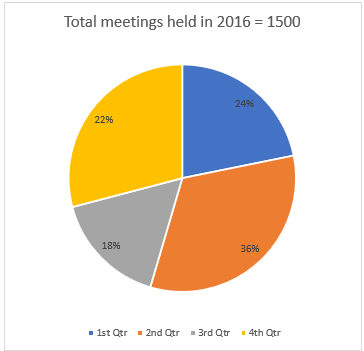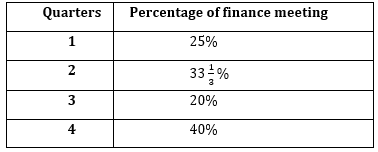Q6. If total management meetings held in 3rd quarter is 40% more than total expenditure meetings held in that quarter and total management meetings held in 4th quarter is 20% more than total Expenditure meetings held in that quarter, then find difference between total management meetings held in 3rd & 4th quarter?
(a) 14
(b) 18
(c) 16
(d) 12
(e) 20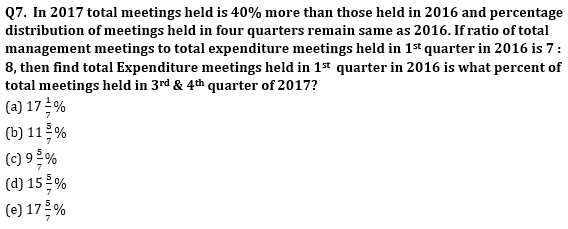Q8. If total expenditure meetings held in 2nd quarter is 40% less than that of total management meetings held in that quarter, then find total expenditure meetings held in 2nd quarter is what percent less than total management & expenditure meetings held in 1st quarter?
(a) 40%
(b) 45%
(c) 25%
(d) 60%
(e) 50%

Q9. If total meetings held in 1st quarter of 2017 is 25% more than total finance meeting held in 1st & 3rd quarter of 2016 together and ratio of finance meeting, management meeting and expenditure meeting held in 1st quarter of 2017 is 9 : 7 : 5, then find ratio of total management meeting held in 1st quarter of 2017 to total finance meeting held in 2nd quarter of 2016?
(a) 1: 4
(b) 1 : 2
(c) 1 : 5
(d) 1 : 3
(e) None of these

Q10. Total management meetings held in 1st , 2nd & 4th quarter is 25%, 40% & 20% more than total expenditure meetings held in these quarter respectively. If total management & expenditure meetings held in 3rd quarter is equal, then find average number of management meetings held in these four quarters of 2016?
(a) 142
(b) 152
(c) 144
(d) 148
(e) 140

Solutions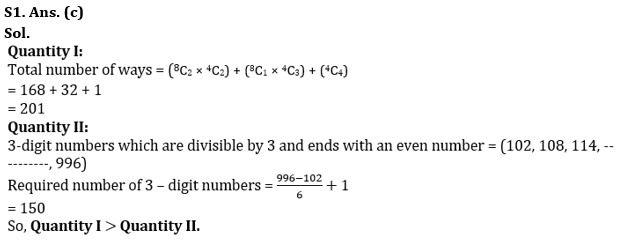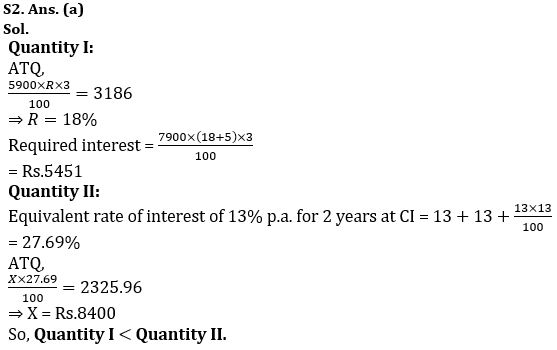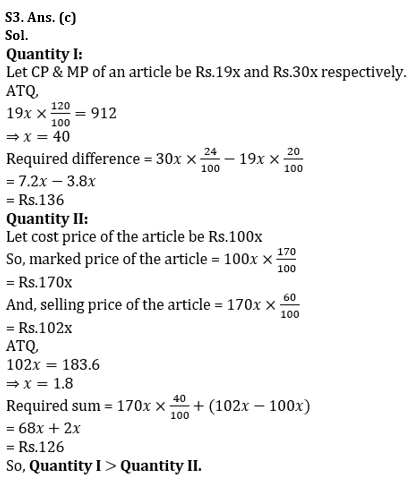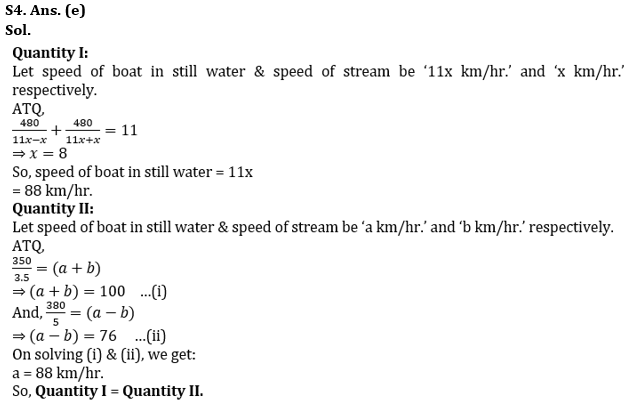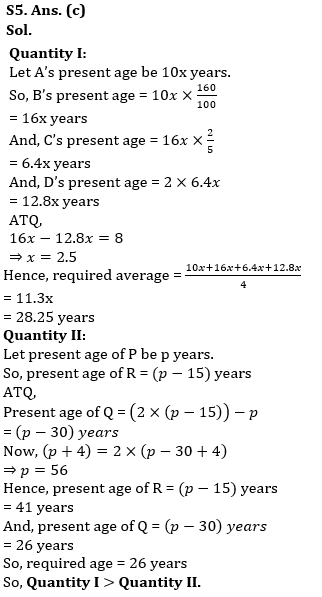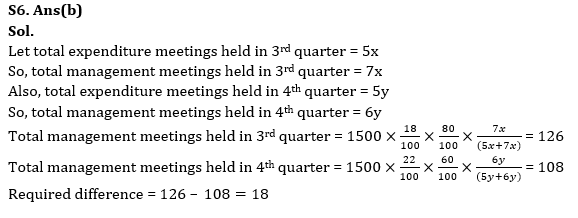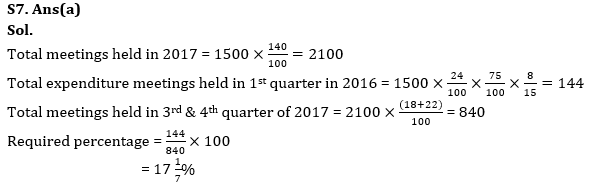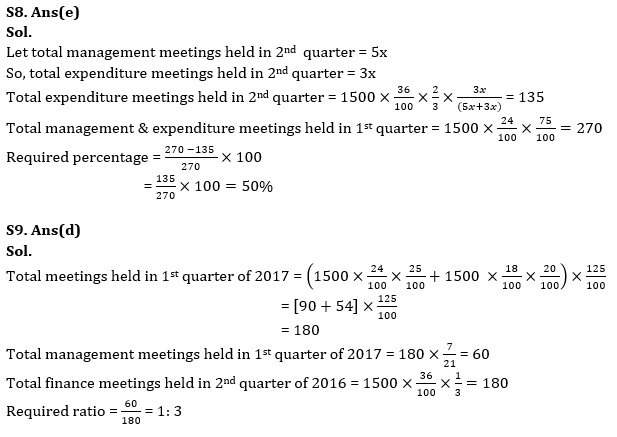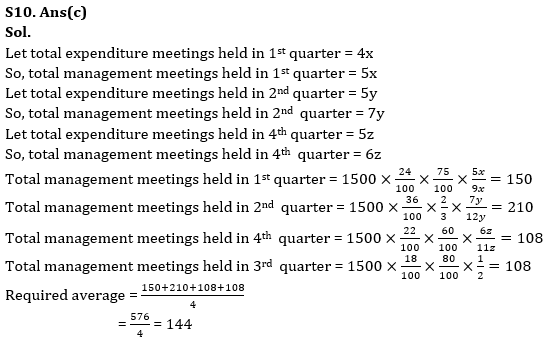## FAQs

### How many sections are there in the RBI Grade B Phase 1 Exam?

How many sections are there in the RBI Grade B Phase 1 Exam?

#### Congratulations!Union Budget 2023-24: Free PDF